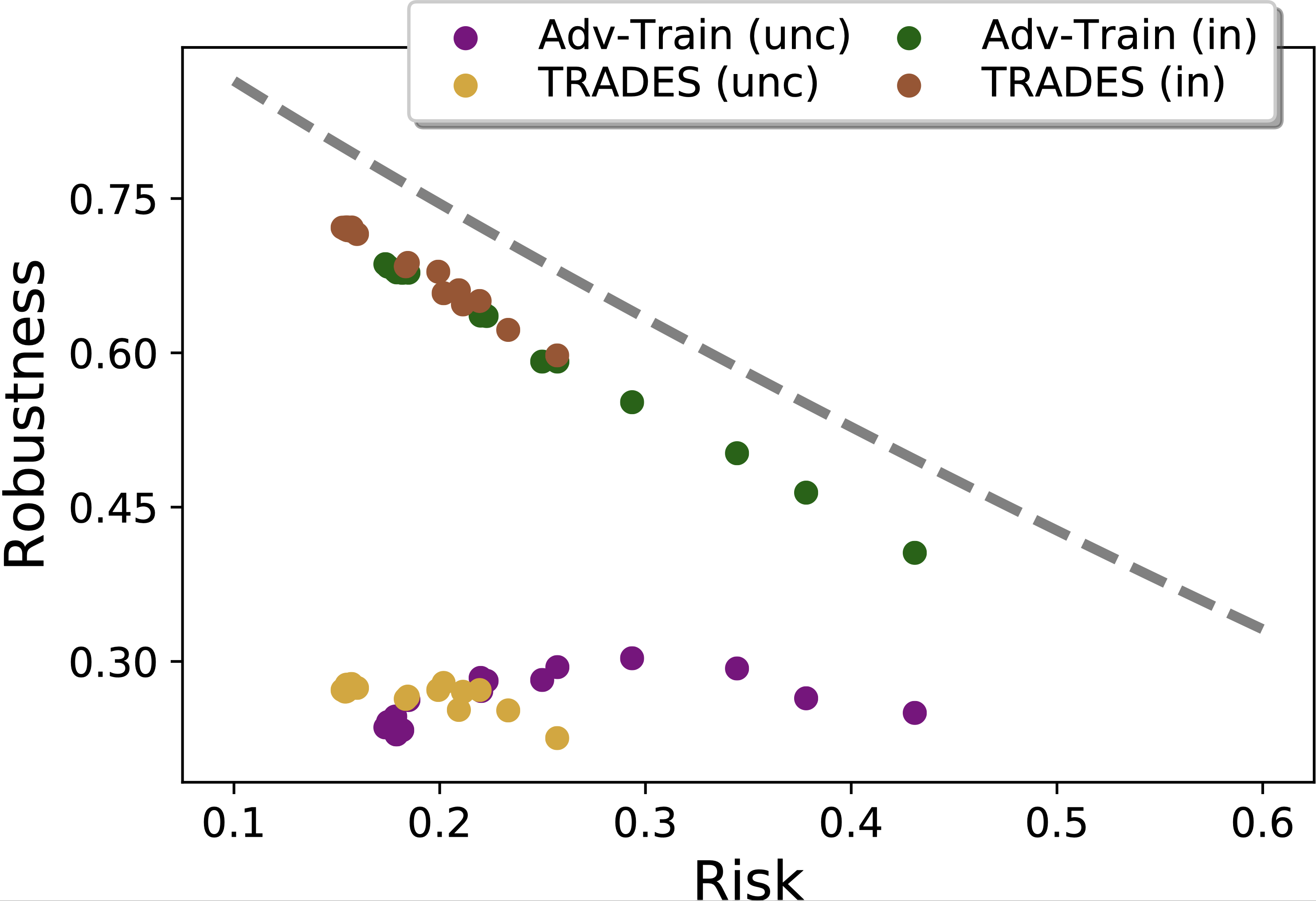# Intrinsic Robustness using Conditional GANs

The video of Xiao’s presentation for AISTATS 2020 is now available: Understanding the Intrinsic Robustness of Image Distributions using Conditional Generative Models

Starting with Gilmer et al. (2018), several works have demonstrated the inevitability of adversarial examples based on different assumptions about the underlying input probability space. It remains unclear, however, whether these results apply to natural image distributions. In this work, we assume the underlying data distribution is captured by some conditional generative model, and prove intrinsic robustness bounds for a general class of classifiers, which solves an open problem in Fawzi et al. (2018). Building upon the state-of-the-art conditional generative models, we study the intrinsic robustness of two common image benchmarks under l2 perturbations, and show the existence of a large gap between the robustness limits implied by our theory and the adversarial robustness achieved by current state-of-the-art robust models.Comparisons between the theoretical intrinsic robustness bound and the empirically estimated unconstrained (unc)/in-distribution (in) adversarial robustness under l2 for ImageNet10 (&epsilon = 3.0). The dotted curve line represents the theoretical bound on intrinsic robustness with horizontal axis denoting the different choice of α. (See paper for details and results in other settings.)

Paper: Xiao Zhang, Jinghui Chen, Quanquan Gu, David Evans. Understanding the Intrinsic Robustness of Image Distributions using Conditional Generative Models), AISTATS 2020. arXiv